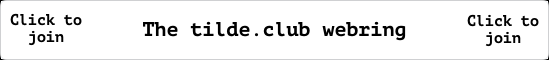back

# Power Electronics - Power Semiconductor and Diodes

A diode acts as a switch, and can help us perform various tasks. Similarly, a power diode exists which is akin to the p-n junction signal diodes but has much higher power, current and voltage handling capabilities. The frequency response of such power diodes is lesser than that of signal diodes(which we will see soon).

## Semiconductor basics and diode characteristics

A power diode, or any diode, is a two terminal device. The terminals are called anode, or cathode. The diode is made by the junction of a p-type material, which has “holes” as it’s majority charge charrier, with n-type material, which has electrons as it’s majority charge carrier.

When the potential of the anode is positive with respect to the cathode, the diode is in forward bias and it conducts. Whereas when the potential of the anode is negative as compared to the cathode, the diode is reverse biased.

The V-I characteristics of a diode can be represented by the schockley diode equation:

$$\begin{equation} \large{I_{D} = I_{S} (E^{V_D/{nV_T}} - 1)} \end{equation}$$

where,

\begin{aligned} & I_D \text{ is the diode current} \\ & I_{S} \text{ is the reverse saturation current} \\ & V_D \text{ is the diode voltage drop} \\ & n \text{ is the ideality factor} \\ & V_T \text{ is the thermal voltage, which can be found out by} \\ & V_T = \frac{kT}{q} \\ & \text{k is the boltzmann constant, T is the temperature of the diode and q is the charge of an electron} & \end{aligned}

### Reverse recovery current

The current in forward bias is due to the net effect of the majority and minority carriers. When a diode is in forward bias, and the diode current falls down to zero, the diode continues to conduct some current as there are minority charge carriers still present in the p-n junction. They need time to recombine with opposite charges and diffuse. This time required is termed as the reverse recovery time.

Reverse recovery time $t_{RR}$ can be defined as the time interval from the instant the diode current passes through zero during change-over from forward bias till the reverse current has reached 25% of it’s peak value.

Reverse recovery charge $Q_{RR}$ is defined as the amount of charge that flows due to the changeover from forward conduction to reverse blocking conduction.

\begin{align} Q_{RR} & \approx \frac{1}{2} I_{RR} t_{RR} \\ I_{RR} & \approx \sqrt{2Q_{RR} \frac{di}{dt}} \end{align}

## Types of Power diodes

An ideal power diode will have negligible reverse recovery time, but the manufacture of such diodes is unfeasable and the effect of the recovery time depends on the application. Therefore, depeding on the recovery time and on the manufacturing techniques the power diodes are divided into three types:

1. General Purpose diodes

• They have high recovery time, and thus are used in low-speed operations.
• Used in line frequency applications, like rectifiers.
2. Fast recovery diodes

• They have very low recovery time.
• Used in dc-dc or dc-ac converters, high speed applications.Click for the [ Random page ]